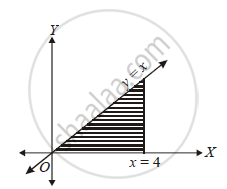# Triangle Bounded by the Lines y = 0, y = x and x = 4 is Revolved About the X-axis. Find the Volume of The Solid of Revolution. - Mathematics and Statistics

triangle bounded by the lines y = 0, y = x and x = 4 is revolved about the X-axis. Find the volume of the solid of revolution.

#### Solution

Given line is y = x

V=piint_0^4 y^2dx

=pi int_0^4x^2dx

=pi/3[x^3]_0^4

=(64pi)/3 cubic units.Concept: Area of the Region Bounded by a Curve and a Line
Is there an error in this question or solution?